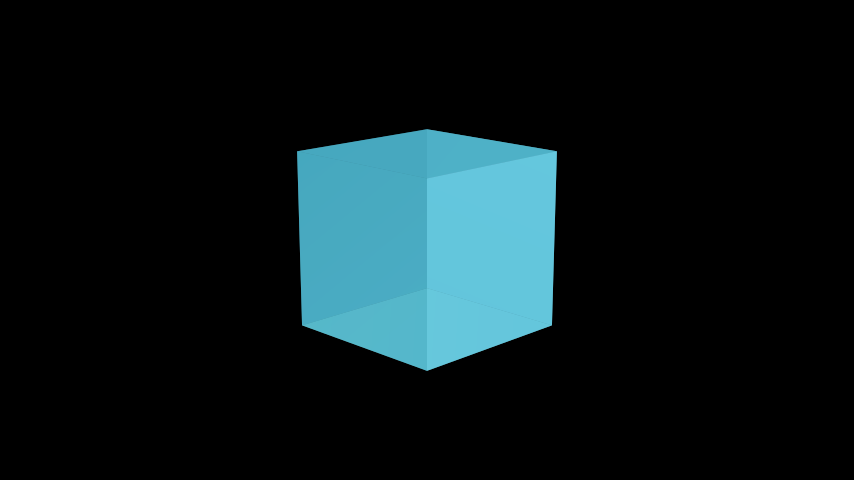# Cube#

Qualified name: `manim.mobject.three\_d.three\_dimensions.Cube`

class Cube(side_length=2, fill_opacity=0.75, fill_color='#58C4DD', stroke_width=0, **kwargs)[source]#

Bases: `VGroup`

A three-dimensional cube.

Parameters
• side_length (float) – Length of each side of the `Cube`.

• fill_opacity (float) – The opacity of the `Cube`, from 0 being fully transparent to 1 being fully opaque. Defaults to 0.75.

• fill_color (Color) – The color of the `Cube`.

• stroke_width (float) – The width of the stroke surrounding each face of the `Cube`.

Examples

Example: CubeExample```from manim import *

class CubeExample(ThreeDScene):
def construct(self):
self.set_camera_orientation(phi=75*DEGREES, theta=-45*DEGREES)

axes = ThreeDAxes()
cube = Cube(side_length=3, fill_opacity=0.7, fill_color=BLUE)
```

Methods

 `generate_points` Creates the sides of the `Cube`. `init_points` Creates the sides of the `Cube`.

Attributes

 `animate` Used to animate the application of any method of `self`. `animation_overrides` `color` `depth` The depth of the mobject. `fill_color` If there are multiple colors (for gradient) this returns the first one `height` The height of the mobject. `n_points_per_curve` `sheen_factor` `stroke_color` `width` The width of the mobject.
generate_points()[source]#

Creates the sides of the `Cube`.

Return type

None

init_points()#

Creates the sides of the `Cube`.

Return type

None